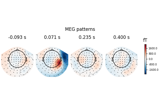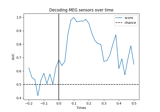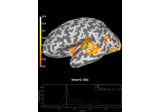# mne.decoding.LinearModel#

class mne.decoding.LinearModel(model=None)[source]#

Compute and store patterns from linear models.

The linear model coefficients (filters) are used to extract discriminant neural sources from the measured data. This class computes the corresponding patterns of these linear filters to make them more interpretable .

Parameters:
modelobject | `None`

A linear model from scikit-learn with a fit method that updates a `coef_` attribute. If None the model will be LogisticRegression.

Notes

New in v0.10.

References

Attributes:
filters_`ndarray`, shape ([n_targets], n_features)

If fit, the filters used to decompose the data.

patterns_`ndarray`, shape ([n_targets], n_features)

If fit, the patterns used to restore M/EEG signals.

Methods

 `fit`(X, y, **fit_params) Estimate the coefficients of the linear model. `get_params`([deep]) Get parameters for this estimator. `set_params`(**params) Set the parameters of this estimator.
fit(X, y, **fit_params)[source]#

Estimate the coefficients of the linear model.

Save the coefficients in the attribute `filters_` and computes the attribute `patterns_`.

Parameters:
X`array`, shape (n_samples, n_features)

The training input samples to estimate the linear coefficients.

y`array`, shape (n_samples, [n_targets])

The target values.

**fit_params`dict` of `str` -> object

Parameters to pass to the fit method of the estimator.

Returns:
selfinstance of `LinearModel`

Returns the modified instance.

Examples using `fit`:Linear classifier on sensor data with plot patterns and filters

Linear classifier on sensor data with plot patterns and filters
get_params(deep=True)[source]#

Get parameters for this estimator.

Parameters:
deepbool, optional

If True, will return the parameters for this estimator and contained subobjects that are estimators.

Returns:
params`dict`

Parameter names mapped to their values.

set_params(**params)[source]#

Set the parameters of this estimator.

The method works on simple estimators as well as on nested objects (such as pipelines). The latter have parameters of the form `<component>__<parameter>` so that it’s possible to update each component of a nested object.

Parameters:
**params`dict`

Parameters.

Returns:
instinstance

The object.

## Examples using `mne.decoding.LinearModel`#Decoding (MVPA)

Decoding (MVPA)Decoding source space data

Decoding source space dataLinear classifier on sensor data with plot patterns and filters

Linear classifier on sensor data with plot patterns and filters